Next: Discontinuous Opening Up: Fluid Section Types: Open Previous: Weir slope   Contents

### Discontinuous Slope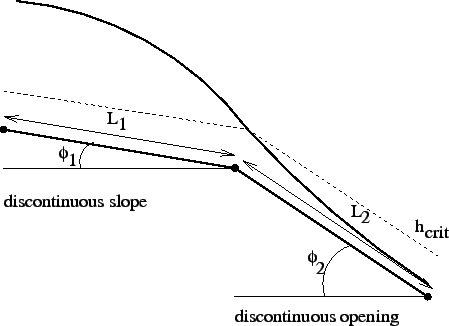The discontinuous slope is the upstream element of a pair of boundary elements simulating a change in slope. The corresponding downstream element is the discontinuous opening. Both are illustrated in Figure 125. The following constants have to be specified on the line beneath the *FLUID SECTION,TYPE=CHANNEL DISCONTINUOUS SLOPE card:

• the width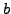•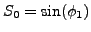(Figure 125; if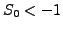the slope is calculated from the coordinates of the end nodes belonging to the element)
• the length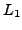(Figure 125; if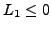the length is calculated from the coordinates of the end nodes belonging to the element)
• the trapezoid angle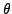• the grain diameter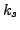for the White-Colebrook law or the Manning constant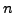for the Manning law (in the latter case the user has to specify the parameter MANNING on the *FLUID SECTION card)
• the number of the upstream reference element
• not used
• the number of the downstream discontinuous opening element
• not used

The lengthis typically small compared to the length of the adjacent channel branches.

Notice that a discontinuous slope element generally has upstream channel elements attached to it. Therefore, it is always mandatory to specify an upstream reference element.

Example files: channel6.Next: Discontinuous Opening Up: Fluid Section Types: Open Previous: Weir slope   Contents
guido dhondt 2018-12-15